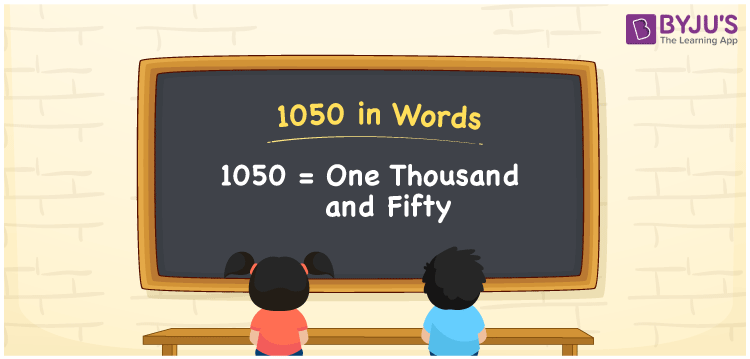# 1050 in Words

We can write 1050 in words as One thousand and fifty. We can convert 1050 into words with the help of a place value chart effortlessly. If you paid Rs. 1050 bill in a restaurant, you can say, “I paid One thousand and fifty rupees bill at the restaurant”. In this article, you will learn how to write the cardinal number 1050 in words and some facts about 1050.

 1050 in words One thousand and fifty One thousand and fifty in numerical form 1050

## 1050 in English Words

We generally write numbers in words using the English alphabet. Thus, we can read 1050 in English as “One thousand and fifty”.## How to Write 1050 in Words?

We can convert 1050 into words with the help of a place value chart. For this, we need to make a four-column chart as shown below, since 1050 has four digits.

 Thousands Hundreds Tens Ones 1 0 5 0

Thus, we can write the expanded form of 1050 using the above table as:

1 × Thousand + 0 × Hundred + 5 × Ten + 0 × One

= 1 × 1000 + 0 × 100 + 5 × 10 + 0 × 1

= 1000 + 50

= One thousand + Fifty

= One thousand and fifty

Therefore, 1050 in words = One thousand and fifty.

1050 is a natural number that precedes 1051 and succeeds 1049.

1050 in words – One thousand and fifty

Is 1050 an odd number? – No

Is 1050 an even number? – Yes

Is 1050 a perfect square number? – No

Is 1050 a perfect cube number? – No

Is 1050 a prime number? – No

Is 1050 a composite number? – Yes

## Frequently Asked Questions on 1050 in Words

Q1

### How do you write 1050 in words?

We can write 1050 in English words as One thousand and fifty.
Q2

### What number is One thousand and fifty?

The number 1050 is spelt as One thousand and fifty, i.e. 1050 is One thousand and fifty.
Q3

### How do you write 1050 in words on a cheque?

On a cheque, we generally write 1050 in words as One thousand and fifty rupees only.
Test your Knowledge on 1050 in Words